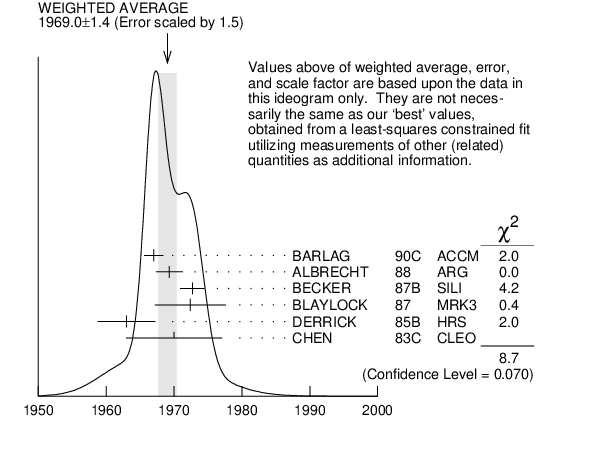# ${{\boldsymbol D}_{{s}}^{\pm}}$ MASS INSPIRE search

The fit includes ${{\mathit D}^{\pm}}$, ${{\mathit D}^{0}}$, ${{\mathit D}_{{s}}^{\pm}}$, ${{\mathit D}^{*\pm}}$, ${{\mathit D}^{*0}}$, ${{\mathit D}_{{s}}^{*\pm}}$, ${{\mathit D}_{{1}}{(2420)}^{0}}$, ${{\mathit D}_{{2}}^{*}{(2460)}^{0}}$, and ${{\mathit D}_{{s1}}{(2536)}^{\pm}}$ mass and mass difference measurements. Measurements of the ${{\mathit D}_{{s}}^{\pm}}$ mass with an error greater than 10 MeV are omitted from the fit and average. A number of early measurements have been omitted altogether.
VALUE (MeV) EVTS DOCUMENT ID TECN  COMMENT
$\bf{ 1968.34 \pm0.07}$ OUR FIT
$\bf{ 1969.0 \pm1.4}$ OUR AVERAGE  Error includes scale factor of 1.5.
$1967.0$ $\pm1.0$ $\pm1.0$ 54
 1990 C
ACCM ${{\mathit \pi}^{-}}{}^{}\mathrm {Cu}$ 230 GeV
$1969.3$ $\pm1.4$ $\pm1.4$
 1988
ARG ${{\mathit e}^{+}}{{\mathit e}^{-}}$ $9.4-10.6$ GeV
$1972.7$ $\pm1.5$ $\pm1.0$ 21
 1987 B
SILI 200 GeV ${{\mathit \pi}},{{\mathit K}},{{\mathit p}}$
$1972.4$ $\pm3.7$ $\pm3.7$ 27
 1987
MRK3 ${{\mathit e}^{+}}{{\mathit e}^{-}}$ $4.14$ GeV
$1963$ $\pm3$ $\pm3$ 30
 1985 B
HRS ${{\mathit e}^{+}}{{\mathit e}^{-}}$ 29 GeV
$1970$ $\pm5$ $\pm5$ 104
 1983 C
CLEO ${{\mathit e}^{+}}{{\mathit e}^{-}}$ $10.5$ GeV
• • • We do not use the following data for averages, fits, limits, etc. • • •
$1968.3$ $\pm0.7$ $\pm0.7$ 290 1
 1988
E691 Photoproduction
$1980$ $\pm15$ 6
 1986
EMUL ${{\mathit \nu}}$ wideband
$1973.6$ $\pm2.6$ $\pm3.0$ 163
 1985 D
ARG ${{\mathit e}^{+}}{{\mathit e}^{-}}$ 10 GeV
$1948$ $\pm28$ $\pm10$ 65
 1984 D
TPC ${{\mathit e}^{+}}{{\mathit e}^{-}}$ 29 GeV
$1975$ $\pm9$ $\pm10$ 49
 1984
TASS ${{\mathit e}^{+}}{{\mathit e}^{-}}$ 14$-$25 GeV
$1975$ $\pm4$ 3
 1984
ACCM hadron${}^{+}$ ${}^{}\mathrm {Be}$ $\rightarrow$ ${{\mathit \phi}}{{\mathit \pi}^{+}}$ X
1  ANJOS 1988 enters the fit via ${\mathit m}_{{{\mathit D}_{{s}}^{\pm}}}–{\mathit m}_{{{\mathit D}^{\pm}}}$ (see below).${{\mathit D}_{{s}}^{\pm}}$ mass (MeV)
References:
 BARLAG 1990C
ZPHY C46 563 Measurement of the Masses and Lifetimes of the Charmed Mesons ${{\mathit D}^{0}}$, ${{\mathit D}^{+}}$ and ${{\mathit D}_{{s}}^{-}}$
 ALBRECHT 1988
PL B207 349 Measurement of ${{\mathit D}_{{s}}^{*}}$ $−$ ${{\mathit D}_{{s}}}$ Mass Difference
 ANJOS 1988
PRL 60 897 Measurement of ${{\mathit D}_{{s}}^{+}}$ Decays and Cabibbo Suppressed ${{\mathit D}^{\pm}}$ Decays
 BECKER 1987B
PL B184 277 Lifetime Measurement of ${{\mathit D}_{{s}}^{\pm}}$ Mesons
 BLAYLOCK 1987
PRL 58 2171 Observation of ${{\mathit e}^{+}}$ ${{\mathit e}^{-}}$ $\rightarrow$ ${{\mathit D}_{{s}}^{\pm}}{{\mathit D}}{}^{(*)\mp{}}_{s}$ at $\sqrt {s }$ = 4.14 GeV
 USHIDA 1986
PRL 56 1767 Lifetimes of the Charmed Particles ${{\mathit D}^{\pm}}$, ${{\mathit D}_{{s}}^{-}}$ and ${{\mathit \Lambda}_{{c}}^{+}}$ Produced by Neutrinos
 ALBRECHT 1985D
PL 153B 343 Production and Decay of the ${{\mathit D}_{{s}}}$ Meson in ${{\mathit e}^{+}}{{\mathit e}^{-}}$ Annihilation at 10 GeV Centre of Mass Energy
 DERRICK 1985B
PRL 54 2568 Production of ${{\mathit \phi}}$ and ${{\mathit F}{(1970)}}$ $\rightarrow$ ${{\mathit \phi}}{{\mathit \pi}^{\pm}}$ in ${{\mathit e}^{+}}{{\mathit e}^{-}}$ Annihilation at 29 GeV
 AIHARA 1984D
PRL 53 2465 Evidence for the ${{\mathit F}^{*}}$ Meson
 ALTHOFF 1984
PL 136B 130 Observation of ${{\mathit F}}$ Meson Production in High Energy ${{\mathit e}^{+}}{{\mathit e}^{-}}$ Annihilation
 BAILEY 1984
PL 139B 320 Measurement of Mass and Lifetime of Hadronically Produced Charmed ${{\mathit F}}$ Mesons
 CHEN 1983C
PRL 51 634 Evidence for the $\mathit F$ Meson at 1970 MeV